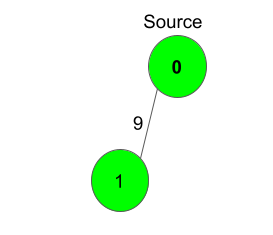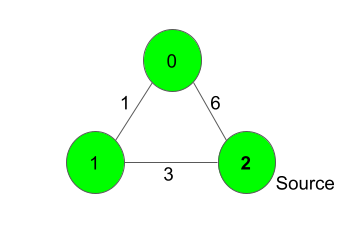XDAYS

:

HOUR

:

MINS

:

SEC

Copied to Clipboard
Implementing Dijkstra Algorithm
Medium Accuracy: 49.0% Submissions: 60987 Points: 4

Given a weighted, undirected and connected graph of V vertices and E edges, Find the shortest distance of all the vertex's from the source vertex S.
Note: The Graph doesn't contain any negative weight cycle.

Example 1:

Input:S = 0
Output:
0 9
Explanation:
The source vertex is 0. Hence, the shortest
distance of node 0 is 0 and the shortest
distance from node 9 is 9 - 0 = 9.


Example 2:

Input:S = 2
Output:
4 3 0
Explanation:
For nodes 2 to 0, we can follow the path-
2-1-0. This has a distance of 1+3 = 4,
whereas the path 2-0 has a distance of 6. So,
the Shortest path from 2 to 0 is 4.
The other distances are pretty straight-forward.


You don't need to read input or print anything. Your task is to complete the function dijkstra()
which takes number of vertices V and an adjacency list adj as input parameters and returns a list of integers, where ith integer denotes the shortest distance of the ith node from Source node. Here adj[i] contains a list of lists containing two integers where the first integer j denotes that there is an edge between i and j and second integer w denotes that the weight between edge i and j is w.

Expected Time Complexity: O(V2).
Expected Auxiliary Space: O(V2).

Constraints:
1
V 1000
0
1 ≤ adj.size() ≤ [ (V*(V - 1)) / 2 ]
0
S < V

We are replacing the old Disqus forum with the new Discussions section given below.

### Editorial

We strongly recommend solving this problem on your own before viewing its editorial. Do you still want to view the editorial?

#### My Submissions: